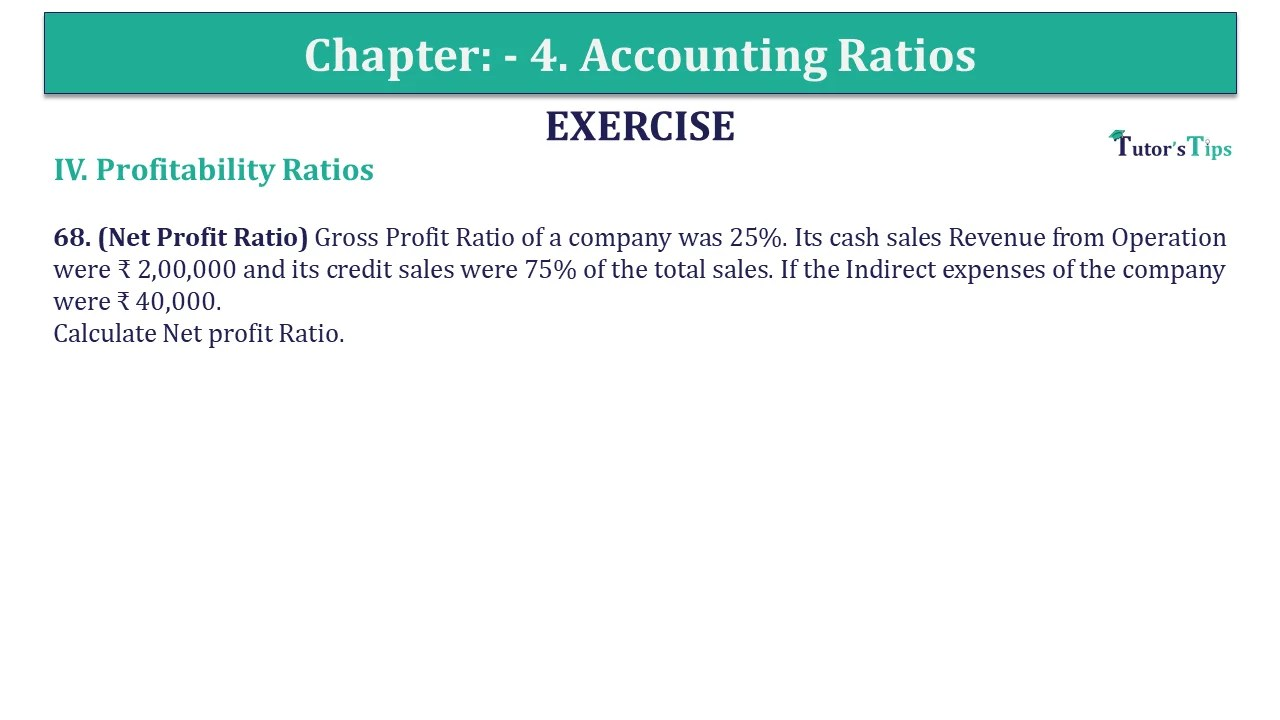# Question 68 Chapter 4 of +2-B – USHA Publication 12 ClassQ-68- CH-4 Book 2 - Usha Pub. +2 Book 2020 - Solution

Question 68 Chapter 4 of +2-B

IV. Profitability Ratios

68. (Net Profit Ratio) The gross Profit Ratio of a company was 25%. Its cash sales Revenue from Operation were ₹ 2,00,000 and its credit sales were 75% of the total sales. If the Indirect expenses of the company were ₹ 40,000.
Calculate Net profit Ratio.

## The solution of Question 68 Chapter 4 of +2-B: –

 Total Sales = Cash Sales + Credit Sales Cash Sales = ₹ 2,00,000 Credit Sales = 75% of Total sales
 Total Sales = 100 X ₹ 2,00,000 25 = ₹ 8,00,000
 Gross Profit = 25 X 8,00,000 100 = ₹ 2,00,000 Net Profit = Gross Profit – Indirect Expenses = ₹ 2,00,000 – ₹ 40,000 = ₹ 1,60,000
 Net Profit Ratio = Net Profit X 100 Net Sales
 = ₹ 1,60,000 X ₹ 1,50,000 ₹ 8,00,000 = 20%

Also, Check out the solved question of previous Chapters: –